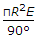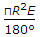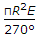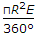# Online Civil Engineering Test - Advanced Surveying TestLoading Test...

Instruction:

• This is a FREE online test. DO NOT pay money to anyone to attend this test.
• Total number of questions : 20.
• Time alloted : 30 minutes.
• Each question carry 1 mark, no negative marks.
• DO NOT refresh the page.
• All the best :-).

1.

The stereo plotting instruments are generally manufactured on the principle of

A.
 optical projectionB.
 optical mechanism projectionC.
 mechanical projectionD.
 all the above.2.

The relief displacement of a minar 72 m high on photograph is 7.2 mm and its top appears 10 cm away from principal point. The flying height of the camera, is

A.
 500 mB.
 1000 mC.
 1500 mD.
 2000 m.3.

Pick up the correct statement from the following. The difference between the longitudes of the places is obtained.

A.
 by subtracting their longitudes if places are in the same hemisphereB.
 by adding their longitudes if places are in the different hemispheresC.
 by subtracting the sum of their longitudes exceeding 180° from 360° if places are in different hemispheresD.
 all the above.4.

The equation which is obtained by multiplying each equation by the coefficient of its un-knowns and by adding the equations thus formed, is known as

A.
 observation equationB.
 conditional equationC.
 normal equationD.
 none of these.5.

In a tropical year, the number of sidereal days are

A.
 one less than mean solar daysB.
 one more than mean solar daysC.
 equal to mean solar daysD.
 none of these.6.

The position of a heavenly body on the celestial sphere can be completely specified by

A.
 its altitude and azimuthB.
 its declination and hour angleC.
 its declination and right ascensionD.
 all the above.7.

Invar tapes used for measuring base lines, is made of nickel-iron alloy containing nickel

A.
 24%B.
 36%C.
 40%D.
 60%.8.

An aerial photograph may be assumed as

A.
 parallel projectionB.
 orthogonal projectionC.
 central projectionD.
 none of these.9.

The foot of the perpendicular on the picture plane through the optical centre of the camera lens, is known as

A.
 isocentreB.
 principal pointC.
 perspective centreD.
 plumb line.10.

If f is the focal length of the camera lens and θ is the angle of tilt, the distance of the plumb point from the principal point will be

A.
 f sin θB.
 f cos θC.
 f tan θD.
 f sec θ.11.

Homologous point is

A.
 photo principal pointB.
 ground principal pointC.
 ground isocentreD.
 photo isocentreE.
 all the above.12.

The difference of parallax for a given difference in elevation is independent of

A.
 focal length of the cameraB.
 overall size of the photo graphsC.
 percentage of overlapD.
 all the above.13.

Homologous points are

A.
 opposite corners of a photographB.
 nodal points of the camera lensC.
 corresponding points on the ground and photographD.
 plumb points of stereo pair of photographs.14.

If E is the spherical excess and R the radius of the earth, the surface area of the triangle, is

A.B.C.D.15.

The point on the photograph where bisector between the vertical line through optical centre of the camera lens and the plate perpendicular meets, is known as

A.
 principal pointB.
 isocentreC.
 plumb pointD.
 perspective centre.16.

The distance between the projection centre and the photograph, is called

A.
 principal distanceB.
 principal lineC.
 isocentric distanceD.
 focal length.17.

Pick up the correct statement from the following :

A.
 the horizontal direction of the pole is called astronomical north.B.
 the angle between the direction of true north and the direction of a survey line is called astronomical bearingC.
 the astronomical bearing is generally called azimuthD.
 all the above.18.

In a spherical triangle ABC, right angled at C, sin b equals

A.
 sin a cos AB.
 cos a sin AC.
 tan a cot AD.
 cot A tan aE.
 none of these.19.

The principal line is the line joining the principal point and

A.
 nadirB.
 isocentreC.
 perspective centreD.
 none of these.20.

The angle between the observer's meridian and declination circle of a heavenly body, is known as

A.
 hour angleB.
 azimuthC.
 right ascensionD.
 declination.